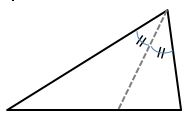# AEPA Mathematics - Middle Grades & Early Secondary (NT105): Practice & Study Guide Final Exam

Free Practice Test Instructions:

Choose your answer to the question and click 'Continue' to see how you did. Then click 'Next Question' to answer the next question. When you have completed the free practice test, click 'View Results' to see your results. Good luck!

#### Question 1 1. Use the integral to find z, the shaded area, in the graph below.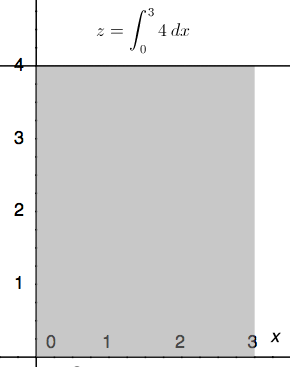#### Question 4 4. What is the complex conjugate of the expression below?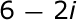#### Question 5 5. What number is left inside the radical after you simplify?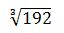#### Question 6 6. Find the perimeter.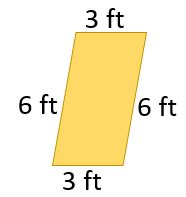#### Question 7 7. Calculate the derivative of g(t).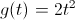#### Question 11 11. What is the average rate of change for the interval with endpoints A and B?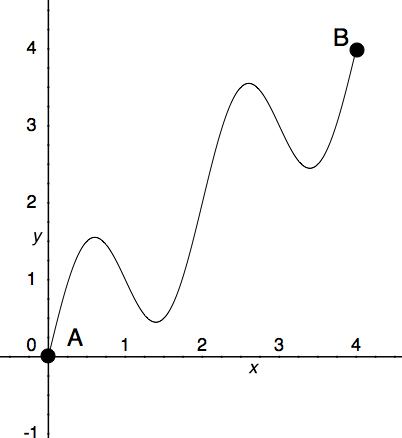#### Question 12 12. If f(x) represents the left part of the black graph and g(x) represents the right part of the black graph, then what piecewise function represents the red graph?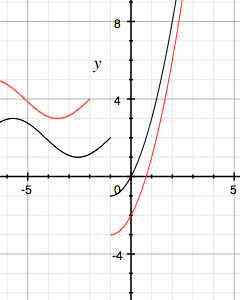#### Question 14 14. What type of line segment is represented by the dotted line in the pictured triangle?